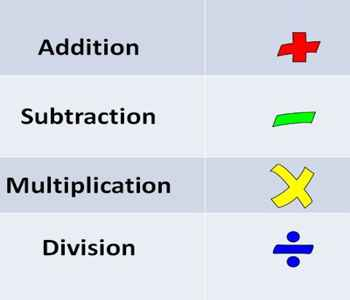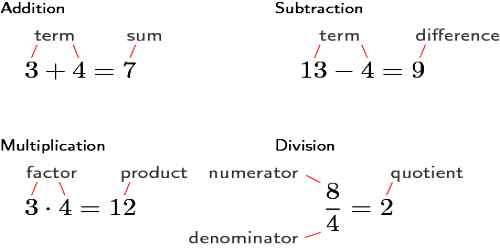Four Basic Operations of Mathematics: Addition, Subtraction, Multiplication and Division

The practice section of this page contains addition, subtraction, multiplication and division problems. Refer to the appropriate lesson if you find that you need extra practice or explanation for any of these operations.

Thus, mastering them is one of the keys to progressing in an understanding of math and, specifically, of algebra.Calculators can be a handy tool for checking answers, but if you rely too heavily on one, you may deprive yourself of the kind of rigorous mental exercises that will help you not just to do math, but to fully understand what you are doing.

Addition is a mathematical operation that explains the total amount of objects when they are put together in a collection.

Subtraction is the arithmetic operation that is the opposite of addition. Subtraction is used when you want to know how many objects are left in the group after you take away a certain amount of objects from that group.

Multiplication: Let’s say we want to add a particular number, such as six, to itself many times. For instance, a worker at a factory may wish to count the number of parts delivered in several boxes. Each box contains six parts, and there are a total of five boxes. To find out how many parts he has, the worker must add the number six to itself five times.

6 + 6 + 6 + 6 + 6

We can find the sum simply by performing the addition several times over. A shortcut, however, is multiplication. Imagine the parts in each of the five boxes laid out in rows, as shown below (we use a square to represent a part).

Division is the fourth basic math operation. Basically, you can say that dividing means splitting objects into equal parts or groups. For example, you have 12 apples that need to be shared equally between 4 people. So, how many apples will each person get? Each person will get 3 apples (12 apples / 4 people = 3 apples per person). The division is the opposite of multiplication: 3 * 4 = 12 and 4 * 3 = 12

12/4 = 3 and 12/3 = 4Example:

Write the missing number: 406 + ? = 104 + 406

Add the following: 318 + 682 = ?

Subtract the following: 845 – 623 = ?

Multiply the following: 73 × 8 = ?